Showing 141 to 150 of 346 results.
0%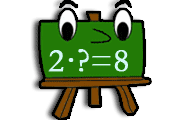## Find the factor

Try to find the requested factors in this game of multiplication. You get a factor and a product and your task is to find the missing product.
Factor Multiplication Product
0%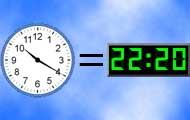## Match clocks

Match clocks that can be both analog and digital. Practice telling time with both types of clocks.
Clock Analog clock Digital clock Numbers
0%## Minecraft math

Count with Minecraft! You will need to multiply, divide and subtract.
Multiplication Division Subtraction Problem solving
0%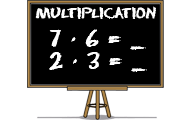## Multiplication

Learn multiplication with this math game for students. Find the product of the factors in all times tables.
Numbers Multiplication Mental calculation
0%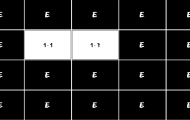## Multiplication memory

Practice multiplication with times tables in this memory game where you should match an expression with the right product.
Multiplication Times tables Mental arithmetic Memory training
0%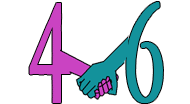## Number bonds

Try to find the number that is a number bond to another number and adds up to the given sum. Practice part-part-whole relationships to the numbers 0-20 and facilitates the important mental calculation
Numbers Addition Mental calculation Number bonds
0%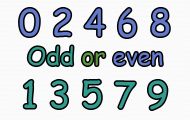## Odd or even numbers

Here you get to practice recognizing even and odd numbers.
Even Odd Numbers
0%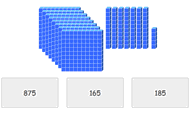## Place value with base ten blocks

Look at the picture and count the hundreds. Then count the number of tens and after that the ones. Sum up and click on the correct number. Practice counting and improve your perception of numbers in t
12 game levels. Kindergarten - Sixth grade
Counting Mental calculation
0%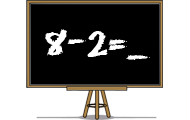## Subtraction

Practice subtraction or minus in a skill training game. Find the difference between two numbers in different levels in this math game.
Numbers Subtraction Mental calculation
0%## Sums of two one-digit numbers

students will be adding one-digit numbers in this game.
Most played
1.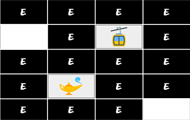Brain training
2.Brain training
3.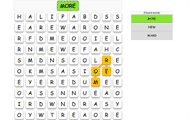English
4.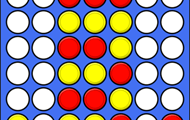Brain training
5.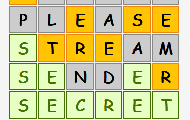English